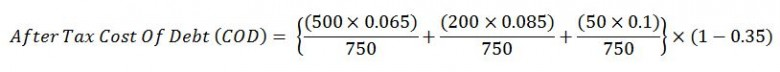# Cost of Debt

Cost of Debt (COD) is an effective rate that a company will pay on it current debt. The Cost of Debt (COD) can be measured in term before or after tax COD however the after tax Cost of Debt (COD) is often used.

After tax Cost of Debt (COD) is mathematically expressed in the equation below;Where;

Di = individual loan amounts

ri = interest rate of the individual loan

Dt = total loans that a company has

t = company corporate tax rate, %

Note: the interest payment on loans is classified as an operating expense and it is a tax deductible.

Example:  Determine the after tax Cost of Debt based on the given information.

James oilfield services issues 500 million \$ in corporate bonds 3 years ago at 6.5 % interest. The company gets a fixed term loan of 200 million \$ at 8.5% interest. Short term debt is 50 million \$ at 10.0% interest. Company corporate tax rate is 35 %.

Total debt (Dt) = 500 + 200 + 50 = 750 million \$After Tax Cost of Debt (COD) = 4.72 %

Reference BookThomas Sowell (2007) Basic economics: A Common Sense Guide to the Economy, New York, New York, USA: Basic Books.

Share the joy
Tagged , , , . Bookmark the permalink.